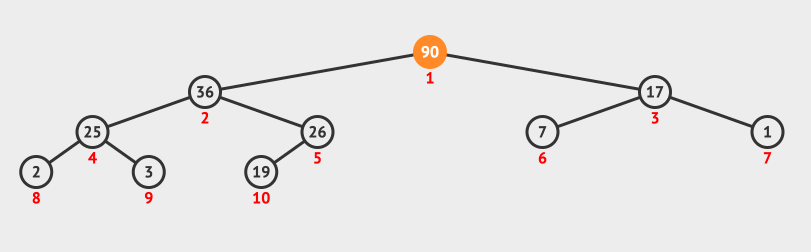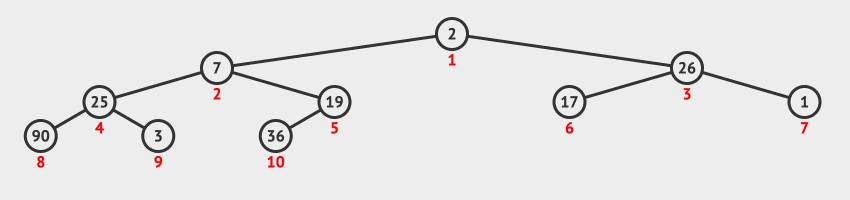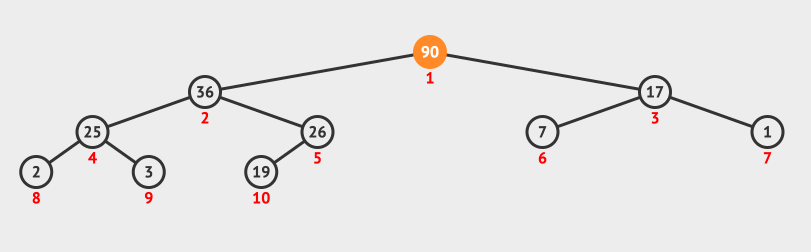07.堆排序

# 堆排序 #

## 1. 堆排序算法思想 #

### 1.1 堆的定义 #

• 大顶堆：根节点值 ≥ 子节点值。
• 小顶堆：根节点值 ≤ 子节点值。

### 1.2 堆排序算法步骤 #

1. 建立初始堆：将无序序列构造成第 1 个大顶堆（初始堆），使得 n 个元素的最大值处于序列的第 1 个位置。
2. 调整堆：交换序列的第 1 个元素（最大值元素）与第 n 个元素的位置。将序列前 n - 1 个元素组成的子序列调整成一个新的大顶堆，使得 n - 1 个元素的最大值处于序列第 1 个位置，从而得到第 2 个最大值元素。
3. 调整堆：交换子序列的第 1 个元素（最大值元素）与第 n - 1 个元素的位置。将序列前 n - 2 个元素组成的子序列调整成一个新的大顶堆，使得 n - 2 个元素的最大值处于序列第 1 个位置，从而得到第 3 个最大值元素。
4. 依次类推，不断交换子序列的第 1 个元素（最大值元素）与当前子序列最后一个元素位置，并将其调整成新的大顶堆。直到子序列剩下一个元素时，排序结束。此时整个序列就变成了一个有序序列。

## 2. 调整堆方法 #

### 2.1 调整堆方法介绍 #

1. 从根节点开始，自上而下地调整节点的位置，使其成为堆积。
1. 判断序号为 i 的节点与其左子树节点（序号为 2 * i）、右子树节点（序号为 2 * i + 1）中值关系。
2. 如果序号为 i 节点大于等于左右子节点值，则排序结束。
3. 如果序号为 i 节点小于左右子节点值，则将序号为 i 节点与左右子节点中值最大的节点交换位置。
2. 因为交换了位置，使得当前节点的左右子树原有的堆积特性被破坏。于是，从当前节点的左右子树节点开始，自上而下继续进行类似的调整。
3. 依次类推，直到整棵完全二叉树成为一个大顶堆。

### 2.2 调整堆方法演示 #1. 交换序列的第 1 个元素 90 与最后 1 个元素 19 的位置，此时当前节点为根节点 19
2. 判断根节点 19与其左右子节点值，因为 17 < 19 < 36，所以将根节点 19 与左子节点 36 互换位置，此时当前节点为根节点 19
3. 判断当前节点 36 与其左右子节点值，因为 19 < 25 < 26，所以将当前节点 19 与右节点 26 互换位置。调整堆结束。

## 3. 建立初始堆方法 #

### 3.1 建立初始堆方法介绍 #

1. 如果原始序列对应的完全二叉树（不一定是堆）的深度为 d，则从 d - 1 层最右侧分支节点（序号为 $\lfloor \frac{n}{2} \rfloor$）开始，初始时令 $i = \lfloor \frac{n}{2} \rfloor$，调用调整堆算法。
2. 每调用一次调整堆算法，执行一次 i = i - 1，直到 i == 1 时，再调用一次，就把原始序列调整为了一个初始堆。

### 3.2 建立初始堆方法演示 #1. 原始序列为 [2, 7, 26, 25, 19, 17, 1, 90, 3, 36]，对应完全二叉树的深度为 3
2. 从第 2 层最右侧的分支节点，也就序号为 5 的节点开始，调用堆调整算法，使其与子树形成大顶堆。
3. 节点序号减 1，对序号为 4 的节点，调用堆调整算法，使其与子树形成大顶堆。
4. 节点序号减 1，对序号为 3 的节点，调用堆调整算法，使其与子树形成大顶堆。
5. 节点序号减 1，对序号为 2 的节点，调用堆调整算法，使其与子树形成大顶堆。
6. 节点序号减 1，对序号为 1 的节点，调用堆调整算法，使其与子树形成大顶堆。
7. 此时整个原始序列对应的完全二叉树就成了一个大顶堆，建立初始堆完毕。

## 4. 堆排序方法完整演示 #1. 原始序列为 [2, 7, 26, 25, 19, 17, 1, 90, 3, 36]，先根据原始序列建立一个初始堆。
2. 交换序列中第 1 个元素（90）与第 10 个元素（2）的位置。将序列前 9 个元素组成的子序列调整成一个大顶堆，此时堆顶变为 36
3. 交换序列中第 1 个元素（36）与第 9 个元素（3）的位置。将序列前 8 个元素组成的子序列调整成一个大顶堆，此时堆顶变为 26
4. 交换序列中第 1 个元素（26）与第 8 个元素（2）的位置。将序列前 7 个元素组成的子序列调整成一个大顶堆，此时堆顶变为 25
5. 以此类推，不断交换子序列的第 1 个元素（最大值元素）与当前子序列最后一个元素位置，并将其调整成新的大顶堆。直到子序列只剩下最后一个元素 1 时，排序结束。此时整个序列变成了一个有序序列，即 [1, 2, 3, 7, 17, 19, 25, 26, 36, 90]

## 5. 堆排序算法分析 #

• 时间复杂度：$O(n \times \log_2 n)$。
• 堆积排序的时间主要花费在两个方面：「建立初始堆」和「调整堆」。
• 设原始序列所对应的完全二叉树深度为 $d$，算法由两个独立的循环组成：
1. 在第 $1$ 个循环构造初始堆积时，从 $i = d - 1$ 层开始，到 $i = 1$ 层为止，对每个分支节点都要调用一次调整堆算法，而一次调整堆算法，对于第 $i$ 层一个节点到第 $d$ 层上建立的子堆积，所有节点可能移动的最大距离为该子堆积根节点移动到最后一层（第 $d$ 层） 的距离，即 $d - i$。而第 $i$ 层上节点最多有 $2^{i-1}$ 个，所以每一次调用调整堆算法的最大移动距离为 $2^{i-1} * (d-i)$。因此，堆积排序算法的第 $1$ 个循环所需时间应该是各层上的节点数与该层上节点可移动的最大距离之积的总和，即：$\sum_{i = d - 1}^1 2^{i-1} (d-i) = \sum_{j = 1}^{d-1} 2^{d-j-1} \times j = \sum_{j = 1}^{d-1} 2^{d-1} \times {j \over 2^j} \le n \sum_{j = 1}^{d-1} {j \over 2^j} < 2n$。这一部分的时间花费为 $O(n)$。
2. 在第 $2$ 个循环中，每次调用调整堆算法一次，节点移动的最大距离为这棵完全二叉树的深度 $d = \lfloor \log_2(n) \rfloor + 1$，一共调用了 $n - 1$ 次调整堆算法，所以，第 $2$ 个循环的时间花费为 $(n-1)(\lfloor \log_2 (n)\rfloor + 1) = O(n \times \log_2 n)$。
• 因此，堆积排序的时间复杂度为 $O(n \times \log_2 n)$。
• 空间复杂度：$O(1)$。由于在堆积排序中只需要一个记录大小的辅助空间，因此，堆积排序的空间复杂度为：$O(1)$。
• 排序稳定性：堆排序是一种 不稳定排序算法

## 6. 堆排序代码实现 #

  1 2 3 4 5 6 7 8 9 10 11 12 13 14 15 16 17 18 19 20 21 22 23 24 25 26 27 28 29 30 31 32 33 34 35 36 37 38 39 40 41 42 43 44 45  class Solution: # 调整为大顶堆 def heapify(self, arr: [int], index: int, end: int): # 根节点为 index，左节点为 2 * index + 1， 右节点为 2 * index + 2 left = index * 2 + 1 right = left + 1 while left <= end: # 当前节点为非叶子结点 max_index = index if arr[left] > arr[max_index]: max_index = left if right <= end and arr[right] > arr[max_index]: max_index = right if index == max_index: # 如果不用交换，则说明已经交换结束 break arr[index], arr[max_index] = arr[max_index], arr[index] # 继续调整子树 index = max_index left = index * 2 + 1 right = left + 1 # 初始化大顶堆 def buildMaxHeap(self, arr: [int]): size = len(arr) # (size - 2) // 2 是最后一个非叶节点，叶节点不用调整 for i in range((size - 2) // 2, -1, -1): self.heapify(arr, i, size - 1) return arr # 升序堆排序，思路如下： # 1. 先建立大顶堆 # 2. 让堆顶最大元素与最后一个交换，然后调整第一个元素到倒数第二个元素，这一步获取最大值 # 3. 再交换堆顶元素与倒数第二个元素，然后调整第一个元素到倒数第三个元素，这一步获取第二大值 # 4. 以此类推，直到最后一个元素交换之后完毕。 def maxHeapSort(self, arr: [int]): self.buildMaxHeap(arr) size = len(arr) for i in range(size): arr, arr[size - i - 1] = arr[size - i - 1], arr self.heapify(arr, 0, size - i - 2) return arr def sortArray(self, nums: List[int]) -> List[int]: return self.maxHeapSort(nums)